﻿ 带有毒素效应功能反应的植物–食植动物模型的脉冲控制研究

# 带有毒素效应功能反应的植物–食植动物模型的脉冲控制研究Impulsive Control of One Plant-Herbivore Model with Toxin-Determined Functional Response

Abstract: In this paper, one impulsive plant-herbivore model with toxin-determined functional response is considered. The trivial periodic solution corresponding to plant extinction, and its local and global asymptotic stability conditions are established. Some dynamical behavior, such as bistability and bifurcation of non-trivial periodic solutions are verified by numerical simulation. The results show the toxin effect does not change the local dynamics of the trivial periodic solutions in the plant- herbivore model with impulsive control, but it changes their global stability conditions. Although impulsive control is feasible in this model, the toxin effect does increase the difficulty of impulsive control.

1. 引言

Holling II型功能反应可以对一般情形下的食草动物和植物的摄食率进行了恰当的描述，但现实中也可能存在这样的情况：由于食植动物个体之间的相互干扰，食植动物种群密度的增加意味着食植动物的摄食率降低   ，这就是Beddington-DeAngelis功能反应被提出的主要原因。实际上，在   中，Beddington-DeAngelis功能反应已经被用来描述植物的毒素效应。本文中研究的带有毒素效应功能反应植物–食植动物脉冲控制的Beddington-DeAngelis (B-D)模型如下所示

$\left\{\begin{array}{l}\begin{array}{l}\stackrel{˙}{x}=rx\left(1-\frac{x}{K}\right)-D\left(x,y\right)y,\\ \stackrel{˙}{y}=\gamma D\left(x,y\right)y-dy,\end{array}\right\}t\ne nT,\\ y\left(n{T}^{+}\right)=y\left(nT\right)+\mu Tt=nT.\end{array}$ (1.1)

$D\left(x,y\right)=B\left(x,y\right)\left(1-\frac{B\left(x,y\right)}{4G}\right),B\left(x,y\right)=\frac{cx}{1+hcx+\beta uy}.$

2. 动力学分析

$\left(\stackrel{¯}{x}\left(t\right),\stackrel{¯}{y}\left(t\right)\right)=\left(0,{y}^{\ast }{\text{e}}^{-d\left(t\text{\hspace{0.17em}}mod\text{\hspace{0.17em}}T\right)}\right)$ (2.1)

${y}^{\ast }=\frac{\mu T}{1-{\text{e}}^{-dT}}.$ (2.2)

$r

$\mu >{\mu }_{1}=\frac{1}{\beta T}\frac{\left(1-{\text{e}}^{-\left(r\beta /c\right)dT}\right)\left(1-{\text{e}}^{-dT}\right)}{{\text{e}}^{-\left(r\beta /c\right)dT}-{\text{e}}^{-dT}}.$ (2.3)

$\left\{\begin{array}{l}\stackrel{˙}{x}=0,\\ \stackrel{˙}{y}=-dy,\\ y\left(n{T}^{+}\right)=y\left(nT\right)+\mu T.\end{array}$ (2.4)

$y\left(\left(n+1\right){T}^{+}\right)=y\left(n{T}^{+}\right){\text{e}}^{-dT}+\mu T,$ (2.5)

${y}^{\ast }=\frac{\mu T}{1-{\text{e}}^{-dT}}.$ (2.6)

$\left\{\begin{array}{l}\stackrel{˙}{\stackrel{˜}{x}}=r\stackrel{˜}{x}\left(1-\stackrel{˜}{x}/K\right)-cD\left(\stackrel{˜}{x},\stackrel{¯}{y}+\stackrel{¯}{y}\right)\left(\stackrel{¯}{y}+\stackrel{˜}{y}\right),\\ \stackrel{˙}{\stackrel{˜}{y}}=\gamma cD\left(\stackrel{˜}{x},\stackrel{¯}{y}+\stackrel{˜}{y}\right)\left(\stackrel{¯}{y}+\stackrel{˜}{y}\right)-d\stackrel{˜}{y}.\end{array}$ (2.7)

$\stackrel{˜}{y}\left(n{T}^{+}\right)=y\left(nT\right)+\mu T+\stackrel{¯}{y}\left(nT\right)-\mu T=\stackrel{˜}{y}\left(nT\right).$

$\left(\begin{array}{c}\stackrel{˜}{x}\left(nT\right)\\ \stackrel{˜}{y}\left(nT\right)\end{array}\right)=\Phi \left(t\right)\left(\begin{array}{c}\stackrel{˜}{x}\left(0\right)\\ \stackrel{˜}{y}\left(0\right)\end{array}\right),$ (2.8)

$\frac{\text{d}\Phi \left(t\right)}{\text{d}t}=A\left(t\right)\Phi \left(t\right).$ (2.9)

$A\left(t\right)=\left(\begin{array}{c}r-\frac{2\stackrel{˜}{x}}{K}-c\frac{\partial D\left(\stackrel{˜}{x},\stackrel{¯}{y}+\stackrel{˜}{y}\right)}{\partial \stackrel{˜}{x}}\left(\stackrel{¯}{y}+\stackrel{˜}{y}\right)\text{\hspace{0.17em}}\text{\hspace{0.17em}}\text{\hspace{0.17em}}\text{\hspace{0.17em}}-c\left(D\left(\stackrel{˜}{x},\stackrel{¯}{y}+\stackrel{˜}{y}\right)-c\left(\stackrel{¯}{y}+\stackrel{˜}{y}\right)\frac{\partial D\left(\stackrel{˜}{x},\stackrel{¯}{y}\right)}{\partial \stackrel{˜}{y}}\right)\\ \frac{\partial D\left(\stackrel{˜}{x},\stackrel{¯}{y}+\stackrel{˜}{y}\right)}{\partial \stackrel{˜}{x}}\left(\stackrel{¯}{y}+\stackrel{˜}{y}\right)\text{\hspace{0.17em}}\text{\hspace{0.17em}}\text{\hspace{0.17em}}\text{\hspace{0.17em}}\text{\hspace{0.17em}}\text{\hspace{0.17em}}\text{\hspace{0.17em}}\text{\hspace{0.17em}}\text{\hspace{0.17em}}\text{\hspace{0.17em}}\text{\hspace{0.17em}}\text{\hspace{0.17em}}\text{\hspace{0.17em}}\text{\hspace{0.17em}}\text{\hspace{0.17em}}\text{\hspace{0.17em}}\text{\hspace{0.17em}}D\left(\stackrel{˜}{x},\stackrel{¯}{y}+\stackrel{˜}{y}\right)+\frac{\partial D\left(\stackrel{˜}{x},\stackrel{¯}{y}+\stackrel{˜}{y}\right)}{\partial \stackrel{˜}{y}}\left(\stackrel{¯}{y}+\stackrel{˜}{y}\right)-d\end{array}\right).$

$\frac{\partial D\left(\stackrel{˜}{x},\stackrel{¯}{y}+\stackrel{˜}{y}\right)}{\partial \stackrel{˜}{x}}=\left(1-\frac{B\left(\stackrel{˜}{x},\stackrel{¯}{y}+\stackrel{˜}{y}\right)}{2G}\right)\frac{\partial B\left(\stackrel{˜}{x},\stackrel{¯}{y}+\stackrel{˜}{y}\right)}{\partial \stackrel{˜}{x}},$

$\frac{\partial D\left(\stackrel{˜}{x},\stackrel{¯}{y}+\stackrel{˜}{y}\right)}{\partial \stackrel{˜}{y}}=\left(1-\frac{B\left(\stackrel{˜}{x},\stackrel{¯}{y}+\stackrel{˜}{y}\right)}{2G}\right)\frac{\partial B\left(\stackrel{˜}{x},\stackrel{¯}{y}+\stackrel{˜}{y}\right)}{\partial \stackrel{˜}{y}},$

$A\left(t\right)=\left(\begin{array}{c}r-\frac{c\stackrel{¯}{y}}{1+\beta y}\text{\hspace{0.17em}}\text{\hspace{0.17em}}\text{\hspace{0.17em}}\text{\hspace{0.17em}}\text{\hspace{0.17em}}\text{\hspace{0.17em}}0\\ \frac{1}{1+\beta y}\text{\hspace{0.17em}}\text{\hspace{0.17em}}\text{\hspace{0.17em}}\text{\hspace{0.17em}}\text{\hspace{0.17em}}\text{\hspace{0.17em}}\text{\hspace{0.17em}}\text{\hspace{0.17em}}-d\end{array}\right),$

$\left(\begin{array}{c}\stackrel{˜}{x}\left(n{T}^{+}\right)\\ \stackrel{˜}{y}\left(n{T}^{+}\right)\end{array}\right)=\left(\begin{array}{cc}1& 0\\ 0& 1\end{array}\right)\left(\begin{array}{c}\stackrel{˜}{x}\left(nT\right)\\ \stackrel{˜}{y}\left(nT\right)\end{array}\right),$ (2.10)

$\Gamma =\left(\begin{array}{cc}1& 0\\ 0& 1\end{array}\right)\Phi \left(T\right)=\Phi \left(T\right).$ (2.11)

${\lambda }_{1}={\text{e}}^{{\int }_{0}^{T}\left(r-\frac{c\stackrel{¯}{y}}{1+\beta \stackrel{¯}{y}}\right)\text{d}t},{\lambda }_{2}={\text{e}}^{-{\int }_{0}^{T}d\text{d}t}.$

${\lambda }_{1}={\text{e}}^{{\int }_{0}^{T}\left(r-\frac{c\stackrel{¯}{y}}{1+\beta \stackrel{¯}{y}}\right)\text{d}t}<1,{\lambda }_{2}={\text{e}}^{{\int }_{0}^{T}\left(-d\right)\text{d}t}<1.$

${\int }_{0}^{T}c{y}^{\ast }{\text{e}}^{-dt}/\left(1+\beta {y}^{\ast }{\text{e}}^{-dt}\right)\text{d}t>rT$

$⇔\left({\text{e}}^{-\left(r\beta /c\right)dT}-{\text{e}}^{-dT}\right)\beta {y}^{\ast }>\left(1-{\text{e}}^{-\left(r\beta /c\right)dT}\right)$ (2.12)

${\text{e}}^{-\left(r\beta /c\right)dT}-{\text{e}}^{-dT}>0$

$r

$\mu >{\mu }_{1}=\frac{\left(1-{\text{e}}^{-\left(r\beta /c\right)dT}\right)\left(1-{\text{e}}^{-dT}\right)}{\beta T\left({\text{e}}^{-\left(r\beta /c\right)dT}-{\text{e}}^{-dT}\right)}.$

$G>\frac{\mathrm{max}\left\{B\left(x,y\right)\right\}}{4}=\underset{x\to \infty }{\mathrm{lim}}\frac{B\left(x,y\right)}{4}=\frac{1}{4h}.$

${D}^{+}V\left(t\right)=\gamma rx\left(1-x/K\right)-dy.$

$\begin{array}{c}{D}^{+}V\left(t\right)+sV\left(t\right)\le \gamma rx\left(1-\frac{x}{K}\right)+s\gamma x\\ =\gamma x\left(r+s\right)-\gamma r{x}^{2}/K\\ \le L,\text{\hspace{0.17em}}L=\frac{\gamma {\left(r+s\right)}^{2}K}{4r}\end{array}$

${D}^{+}V\left(t\right)\le -sV\left(t\right)+L.$

$V\left(n{T}^{+}\right)=\gamma x\left(n{T}^{+}\right)+y\left(n{T}^{+}\right)=V\left(nT\right)+\mu T.$

$V\left(\left(n+1\right){T}^{+}\right)\le V\left(nT\right){\text{e}}^{-sT}+L/s+\mu T$

${V}^{\ast }\left(t\right)\le \frac{L/s+\mu T}{1-{\text{e}}^{-sT}}=B$$n\to \infty$

$\left(\stackrel{¯}{x}\left(t\right),\stackrel{¯}{y}\left(t\right)\right)=\left(0,{y}^{\ast }{\text{e}}^{-d\left(tmodT\right)}\right)$ (2.13)

(H1) $G>\frac{1}{4h}$

(H2) $r<\frac{cM}{\beta }$

(H3) $\mu >{\mu }_{2}=\frac{\left(1+K\right)\left(1-{\text{e}}^{-\left(r\beta /\left(cM\right)\right)dT}\right)\left(1-{\text{e}}^{-dT}\right)}{\beta T\left({\text{e}}^{-\left(r\beta /\left(cM\right)\right)dT}-{\text{e}}^{-dT}\right)}$

$\stackrel{˙}{x}$ 我们将方程(2.7)的第一式除以 $\frac{c\stackrel{˜}{x}}{1+\alpha \stackrel{˜}{x}}$ 并积分得

${\int }_{{t}_{0}}^{t}\frac{\stackrel{˙}{\stackrel{˜}{x}}}{\stackrel{˜}{x}}\text{d}\tau ={\int }_{{t}_{0}}^{t}\left[r/c\left(1-\stackrel{˜}{x}\left(\tau \right)/K\right)-\frac{D\left(\stackrel{˜}{x}\left(\tau \right),\stackrel{¯}{y}\left(\tau \right)+\stackrel{˜}{y}\left(\tau \right)\right)\left(1-\alpha \stackrel{˜}{x}\right)}{c\stackrel{˜}{x}}\left(\stackrel{¯}{y}\left(\tau \right)+\stackrel{˜}{y}\left(\tau \right)\right)\right]\text{d}\tau$

$\begin{array}{c}{\int }_{{t}_{0}}^{t}\frac{\stackrel{˙}{\stackrel{˜}{x}}}{\stackrel{˜}{x}}\text{d}\tau ={\int }_{{t}_{0}}^{\left(⌊\frac{{t}_{0}}{T}⌋+1\right)T}\left[r\left(1-\stackrel{˜}{x}\left(\tau \right)/K\right)-\frac{D\left(\stackrel{˜}{x}\left(\tau \right),\stackrel{¯}{y}\left(\tau \right)+\stackrel{˜}{y}\left(\tau \right)\right)}{\stackrel{˜}{x}}\left(\stackrel{¯}{y}\left(\tau \right)+\stackrel{˜}{y}\left(\tau \right)\right)\right]\text{d}\tau \\ \text{\hspace{0.17em}}\text{\hspace{0.17em}}+{\int }_{\left(⌊\frac{{t}_{0}}{T}⌋+1\right)T}^{\left(⌊\frac{t}{T}⌋\right)}\left[r\left(1-\stackrel{˜}{x}\left(\tau \right)/K\right)-\frac{D\left(\stackrel{˜}{x}\left(\tau \right),\stackrel{¯}{y}\left(\tau \right)+\stackrel{˜}{y}\left(\tau \right)\right)}{\stackrel{˜}{x}}\left(\stackrel{¯}{y}\left(\tau \right)+\stackrel{˜}{y}\left(\tau \right)\right)\right]\text{d}\tau \\ \text{\hspace{0.17em}}\text{\hspace{0.17em}}+{\int }_{\left(⌊\frac{t}{T}⌋\right)T}^{t}\left[r\left(1-\stackrel{˜}{x}\left(\tau \right)/K\right)-\frac{D\left(\stackrel{˜}{x}\left(\tau \right),\stackrel{¯}{y}\left(\tau \right)+\stackrel{˜}{y}\left(\tau \right)\right)}{\stackrel{˜}{x}}\left(\stackrel{¯}{y}\left(\tau \right)+\stackrel{˜}{y}\left(\tau \right)\right)\right]\text{d}\tau \end{array}$

${\int }_{\left(⌊\frac{{t}_{0}}{T}⌋+1\right)T}^{\left(⌊\frac{t}{T}⌋\right)T}\left[r\left(1-\stackrel{˜}{x}\left(\tau \right)/K\right)-\frac{D\left(\stackrel{˜}{x}\left(\tau \right),\stackrel{¯}{y}\left(\tau \right)+\stackrel{˜}{y}\left(\tau \right)\right)}{\stackrel{˜}{x}}\left(\stackrel{¯}{y}\left(\tau \right)+\stackrel{˜}{y}\left(\tau \right)\right)\right]\text{d}\tau \to -\infty$

$\stackrel{˙}{\stackrel{˜}{y}}>-d\stackrel{˜}{y}.$

$\stackrel{¯}{y}\left(\tau \right)+\stackrel{˜}{y}\left(\tau \right)\ge {y}^{\ast }\left({\text{e}}^{-d\left(t\text{\hspace{0.17em}}mod\text{\hspace{0.17em}}T\right)}-{\text{e}}^{-d\left(t-{t}_{0}\right)}\right).$

$\begin{array}{l}{\int }_{\left(⌊\frac{{t}_{0}}{T}⌋+1\right)T}^{\left(⌊\frac{t}{T}⌋\right)T}\left[r\left(1-\stackrel{˜}{x}\left(\tau \right)/K\right)-\frac{D\left(\stackrel{˜}{x}\left(\tau \right),\stackrel{¯}{y}\left(\tau \right)+\stackrel{˜}{y}\left(\tau \right)\right)}{\stackrel{˜}{x}}\left(\stackrel{¯}{y}\left(\tau \right)+\stackrel{˜}{y}\left(\tau \right)\right)\right]\text{d}\tau \\ ={\int }_{\left(⌊\frac{{t}_{0}}{T}⌋+1\right)T}^{\left(⌊\frac{t}{T}⌋\right)T}\left[r\left(1-\stackrel{˜}{x}\left(\tau \right)/K\right)-c\left(1-\frac{B\left(\stackrel{˜}{x}\left(\tau \right),\stackrel{¯}{y}\left(\tau \right)+\stackrel{˜}{y}\left(\tau \right)\right)}{4G}\frac{\stackrel{¯}{y}\left(\tau \right)+\stackrel{˜}{y}\left(\tau \right)}{1+hc\stackrel{˜}{x}+\beta \left(\stackrel{¯}{y}\left(\tau \right)+\stackrel{˜}{y}\left(\tau \right)\right)}\right)\right]\text{d}\tau \\ \le {\int }_{\left(⌊\frac{{t}_{0}}{T}⌋+1\right)T}^{\left(⌊\frac{t}{T}⌋\right)T}\left[r\left(1-\stackrel{˜}{x}\left(\tau \right)/K\right)-cM\frac{\stackrel{¯}{y}\left(\tau \right)+\stackrel{˜}{y}\left(\tau \right)}{1+hc\stackrel{˜}{x}+\beta \left(y\left(\tau \right)+\stackrel{˜}{y}\left(\tau \right)\right)}\right]\text{d}\tau \\ \le {\int }_{\left(⌊\frac{{t}_{0}}{T}⌋+1\right)T}^{\left(⌊\frac{t}{T}⌋\right)T}\left[r\left(1-\stackrel{˜}{x}\left(\tau \right)/K\right)-cM\frac{{y}^{\ast }\left({\text{e}}^{-d\left(t\text{\hspace{0.17em}}mod\text{\hspace{0.17em}}T\right)}-{\text{e}}^{-d\left(t-{t}_{0}\right)}\right)}{1+hc\stackrel{˜}{x}+\beta \left({y}^{\ast }\left({\text{e}}^{-d\left(t\text{\hspace{0.17em}}mod\text{\hspace{0.17em}}T\right)}-{\text{e}}^{-d\left(t-{t}_{0}\right)}\right)\right)}\right]\text{d}\tau \\ \le {\int }_{\left(⌊\frac{{t}_{0}}{T}⌋+1\right)T}^{\left(⌊\frac{t}{T}⌋\right)T}\left[r\left(1-\stackrel{˜}{x}\left(\tau \right)/K\right)-cM\frac{{y}^{\ast }\left({\text{e}}^{-d\left(t\text{\hspace{0.17em}}mod\text{\hspace{0.17em}}\text{​}T\right)}-{\text{e}}^{-d\left(t-{t}_{0}\right)}\right)}{1+hcK+\beta \left({y}^{\ast }\left({\text{e}}^{-d\left(t\text{\hspace{0.17em}}mod\text{\hspace{0.17em}}T\right)}-{\text{e}}^{-d\left(t-{t}_{0}\right)}\right)\right)}\right]\text{d}\tau ,\end{array}$

$\begin{array}{c}M=\mathrm{min}\left\{1-B\left(\stackrel{˜}{x}\left(\tau \right),\stackrel{¯}{y}\left(\tau \right)+\stackrel{˜}{y}\left(\tau \right)\right)/4G\right\}\\ =1-\mathrm{max}\left\{B\left(\stackrel{˜}{x}\left(\tau \right),\stackrel{¯}{y}\left(\tau \right)+\stackrel{˜}{y}\left(\tau \right)\right)/4G\right\}\\ =1-\frac{K}{4G\left(1+\alpha K\right)}.\end{array}$ (2.14)

$\begin{array}{l}{\int }_{\left(⌊\frac{{t}_{0}}{T}⌋+1\right)T}^{\left(⌊\frac{t}{T}⌋\right)T}\left[r\left(1-\stackrel{˜}{x}\left(\tau \right)/K\right)-cM\frac{{y}^{\ast }\left({\text{e}}^{-d\left(t\text{\hspace{0.17em}}mod\text{\hspace{0.17em}}T\right)}-{\text{e}}^{-d\left(t-{t}_{0}\right)}\right)}{1+hc\stackrel{˜}{x}+\beta \left({y}^{\ast }\left({\text{e}}^{-d\left(t\text{\hspace{0.17em}}mod\text{\hspace{0.17em}}T\right)}-{\text{e}}^{-d\left(t-{t}_{0}\right)}\right)\right)}\right]\text{d}\tau \\ ={\sum }_{n=\left(⌊\frac{{t}_{0}}{T}⌋+1\right)}^{⌊\frac{t}{T}⌋-1}I\left(n\right),\end{array}$ (2.15)

$\begin{array}{c}I\left(n\right)={\int }_{\left(⌊\frac{{t}_{0}}{T}⌋+1\right)T}^{\left(⌊\frac{t}{T}⌋\right)T}\left[r\left(1-\stackrel{˜}{x}\left(\tau \right)/K\right)-cM\frac{{y}^{\ast }\left({\text{e}}^{-d\left(t\text{\hspace{0.17em}}mod\text{\hspace{0.17em}}T\right)}-{\text{e}}^{-d\left(t-{t}_{0}\right)}\right)}{1+hc\stackrel{˜}{x}+\beta \left({y}^{\ast }\left({\text{e}}^{-d\left(t\text{\hspace{0.17em}}mod\text{\hspace{0.17em}}T\right)}-{\text{e}}^{-d\left(t-{t}_{0}\right)}\right)\right)}\right]\text{d}\tau \\ =rT+\frac{cM}{\beta d}\cdot \mathrm{ln}\frac{1+hcK+\beta {y}^{\ast }{\text{e}}^{-dT}\left(1-{\text{e}}^{-d\left(nT-{t}_{0}\right)}\right)}{1+hcK+\beta {y}^{\ast }\left(1-{\text{e}}^{-d\left(nT-{t}_{0}\right)}\right)}.\end{array}$ (2.16)

$\underset{n\to +\infty }{\mathrm{lim}}I\left(n\right)=rT+\frac{cM}{\beta d}\cdot \mathrm{ln}\frac{1+hcK+\beta {y}^{\ast }{\text{e}}^{-dT}}{1+hcK+\beta {y}^{\ast }}<0.$

${y}^{\ast }$ 带入解出 $\mu$

$\mu >{\mu }_{2}=\frac{\left(1+K\right)\left(1-{\text{e}}^{-\left(r\beta /\left(cM\right)\right)dT}\right)\left(1-{\text{e}}^{-dT}\right)}{\beta T\left({\text{e}}^{-\left(r\beta /\left(cM\right)\right)dT}-{\text{e}}^{-dT}\right)},$

$\forall t>{t}_{m},\text{\hspace{0.17em}}\gamma cD\left(\stackrel{˜}{x},\stackrel{¯}{y}+\stackrel{˜}{y}\right)<\frac{d}{2},$

$\forall t>{t}_{m},\stackrel{˙}{\stackrel{˜}{y}}\le \gamma cD\left(\stackrel{˜}{x},\stackrel{¯}{y}+\stackrel{˜}{y}\right)\stackrel{¯}{y}-\frac{d}{2}\stackrel{˜}{y}.$

$\underset{G\to +\infty }{\mathrm{lim}}M=1,$

$\underset{G\to +\infty }{\mathrm{lim}}{\mu }_{2}=\frac{\left(1+K\right)\left(1-{\text{e}}^{-\left(r\beta /\left(cM\right)\right)dT}\right)\left(1-{\text{e}}^{-dT}\right)}{\beta T\left({\text{e}}^{-\left(r\beta /\left(cM\right)\right)dT}-{\text{e}}^{-dT}\right)}=\frac{\left(1+K\right)\left(1-{\text{e}}^{-\left(r\beta /c\right)dT}\right)\left(1-{\text{e}}^{-dT}\right)}{\beta T\left({\text{e}}^{-\left(r\beta /c\right)dT}-{\text{e}}^{-dT}\right)}\stackrel{˙}{=}{\mu }_{3}$ (2.17)

3. 数值模拟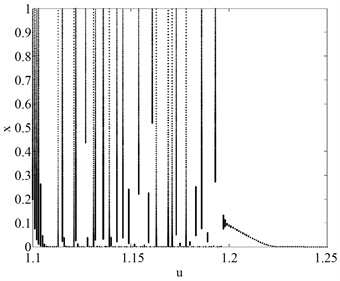(a)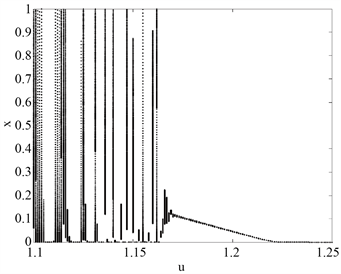(b)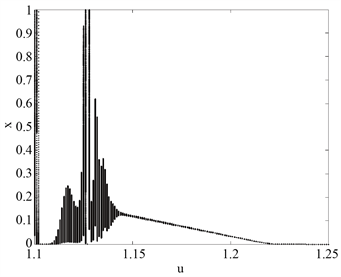(c)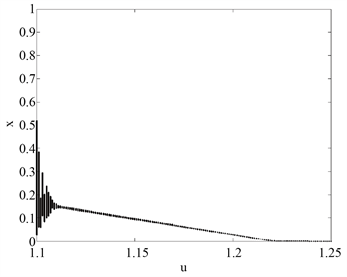(d)

Figure 1. Nontrivial periodic solution bifurcation of the system with respect to μ near the trivial periodic solution with different G. X-label is the parameter μ. Y-label is the population of plant. The critical value of the local asymptotic stability condition of the trivial periodic solution are is μ = 1.22. (a) The window of nontrivial periodic solutions is $\mu \in \left[1.2,1.22\right]$ when G = 0.6; (b) The region of nontrivial periodic solutions is $\mu \in \left[1.17,1.22\right]$ when $G=1$ ; (c) The window of nontrivial periodic solutions is $\mu \in \left[1.14,1.22\right]$ when G = 2. (d)The window of nontrivial periodic solutions is $\mu \in \left[1.12,1.22\right]$ when $G=+\infty$. With the increase of G, the region of nontrivial periodic solutions becomes larger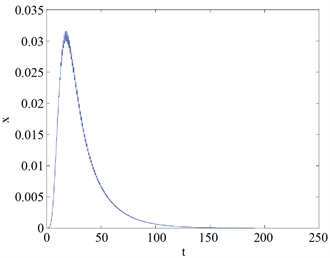(a)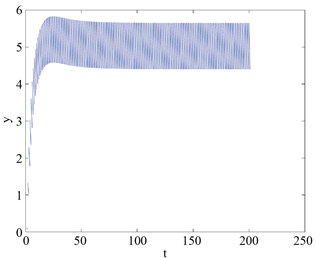(b)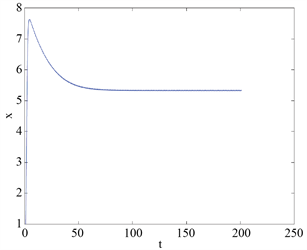(c)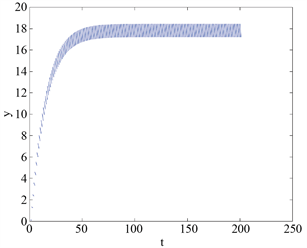(d)

Figure 2. Time plots showing the bistability in system when $\mu =1.25$. (a) (b) The trivial periodic solution when initial value is (0.001,0.1); (c) (d) The non-trivial periodic solution when initial value is (1, 0.1). The system has two stable periodic solutions, one is trivial and the other is nontrivial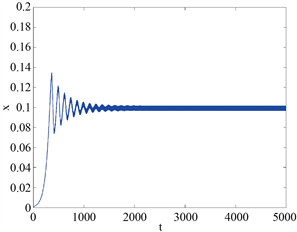(a)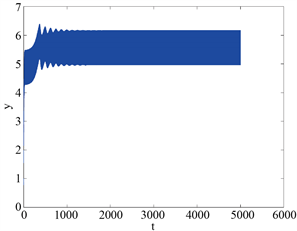(b)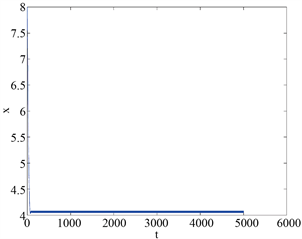(c)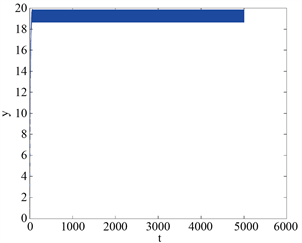(d)

Figure 3. Time plots showing the bistability in system when $\mu =1.2$. (a) (b) The trivial periodic solution when initial value is (0.001, 0.1); (c) (d) The non-trivial periodic solution when initial value is (4, 0.1). The system has two stable non-trivial periodic solutions

4. 结论

NOTES

*通讯作者。

 Feng, Z. and DeAngelis Donald, L. (2017) Mathematical Models of Plant-Herbivore Interactions. CRC Press, Boca Raton.

 Liu, R., Feng, Z., Zhu, H., et al. (2008) Bifurcation Analysis of a Plant-Herbivore Model with Toxin-Determined Functional Response. Journal of Differential Equations, 245, 442-467.
https://doi.org/10.1016/j.jde.2007.10.034

 Feng, Z., Qiu, Z., Liu, R., et al. (2011) Dynamics of a Plant-Herbivore-Predator System with Plant-Toxicity. Mathematical Biosciences, 229, 190-204.
https://doi.org/10.1016/j.mbs.2010.12.005

 Zhang, C.H. and Li, Z.Z. (2014) Dynamics in a Diffusive Plant-Herbivore Model with Toxin-Determined Functional Response. Computers and Mathematics with Applications, 67, 1439-1449.
https://doi.org/10.1016/j.camwa.2014.02.019

 Wang, X., Tao, Y.D. and Song, X.Y. (2010) A Delayed HIV-1 Infection Model with Beddington-DeAngelis Function Response. Nonlinear Dynamics, 62, 67-72.
https://doi.org/10.1007/s11071-010-9699-1

 Tripathi, J.P., Abbas, S. and Thakur, M. (2015) Dynamical Analysis of a Prey-Predator Model with Beddington- DeAngelis Type Function Response Incorporating a Prey Refuge. Nonlinear Dynamics, 80, 177-196.
https://doi.org/10.1007/s11071-014-1859-2

 Negi, K. and Gakkhar, S. (2007) Dynamics in a Beddington-DeAngelis Prey-Predator System with Impulsive Harvesting. Ecological Modelling, 206, 421-430.
https://doi.org/10.1016/j.ecolmodel.2007.04.007

 Wang, Y.S., Wu, H. and Wang, S.K. (2020) Persistence of Pollination Mutualisms under Pesticides. Applied Mathematical Modelling, 77, 861-880.
https://doi.org/10.1016/j.apm.2019.08.009

 Fishman, M.A. and Hadany, L. (2010) Plant-Pollinator Population Dynamics. Theoretical Population Biology, 78, 270- 277.
https://doi.org/10.1016/j.tpb.2010.08.002

 Wang, S. and Huang, Q.D. (2015) Bifurcation of Nontrivial Periodic Solutions for a Beddington-DeAngelis Interference Model with Impulsive Biological Control. Applied Mathematical Modelling, 39, 1470-1479.
https://doi.org/10.1016/j.apm.2014.09.011

 Nundloll, S., Mailleret, L. and Grognard, F. (2010) Two Models of Interfering Predators in Impulsive Biological Control. Journal of Biological Dynamics, 4, 102-114.
https://doi.org/10.1080/17513750902968779

Top